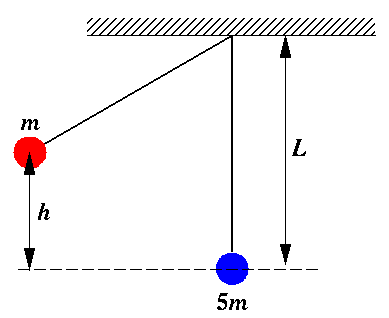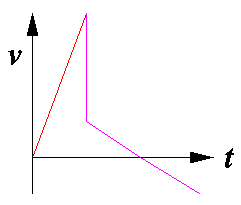*echo Physics Practice for MCAT Directions: Select the best possible answer from the choices A-D. Guessing will not (on average) reduce your score, but if you have no idea how to work a problem, leave it blank and I'll give you the expected ¼ point.
*set show 1 *let time0=secnds *hide time0 e12.6

This is a timed test. Your start time is: *write int(time0/3600) 'i2,1h:,\$' *write (time0-int(time0/3600)*3600)/60 'i2.2'

SHOW= (SHOW=4 is a good start)

## Ballistic PendulumA red sphere (of mass m) and a blue sphere (of mass 5m) are attached to the ceiling by massless strings of identical length forming twin pendulums of length L. The red sphere is drawn to the left so that its center of mass has been raised a distance h and is then released. As the red sphere moves back through equilibrium it hits and adheres to the blue sphere. The combined system swings to the right, eventually reaching some maximum height before returning to equilibrium.

*let seed=pid*secnds *if (ran.gt..5) then

1. How much work did gravity do on the red sphere when it was moved from equilibrium to its release point (as displayed above).
1. mgh
2. -mgh
3. ½ mgh
4. cannot be determined
```A B C D
```
*else
2. How much work had to be done to move the red sphere from equilibrium to its release point (as displayed above).
1. mgh
2. -mgh
3. ½ mgh
4. cannot be determined
```A B C D
```
*endif

*if (ran.gt..5) then

3. What was the speed of the red sphere just before its collision?
1. (2gh)½
2. (gh)½
3. g(L2-h2)½
4. ½ gh2
```A B C D
```
*else
4. If the post-collision speed of the combined spheres is initially v, what maximum height will be achieved?
1. v2/(2gL)½
2. mgh
3. v2/g
4. v2/(2g)
```A B C D
```
*endif

*if (ran.gt..5) then

5. What was the speed of the red sphere just after its collision?
1. (1/2) its speed before the collision
2. (1/5) its speed before the collision
3. (2/5) its speed before the collision
4. (1/6) its speed before the collision
```A B C D
```
*else
6. If the collision were elastic rather than inelastic how would the post-elastic-collision speeds compare to the post-inelastic-collision speed?
1. The red sphere would be moving faster, the blue slower.
2. The red sphere would be moving slower, the blue faster.
3. Both red and blue would be moving faster than the post-inelastic-collision speed.
4. Neither red and blue would be moving faster than the post-inelastic-collision speed.
```A B C D
```
*endif

*if (ran.gt..5) then

7. Compare the time required for the red sphere to move from its release point to the collision point (tred) to the time for the combined spheres to move from the collision point to the point of maximum height (tboth).
1. tred < tboth; the time differential gets larger as h gets bigger.
2. tred > tboth; the time differential gets smaller as h gets bigger.
3. tred and tboth are quite similar as long as h<<L
4. tred and tboth are both proportional to h½
```A B C D
```
*else
8. Which graph best represents the horizontal velocity of the red sphere as a function of time?

ABCD```A B C D
```
*endif

*if (ran.gt..5) then

9. In this problem:
1. momentum and energy are always conserved.
2. potential energy is converted into kinetic energy, but their total is conserved.
3. angular momentum is conserved since the string exerts no torque.
4. momentum is conserved only during the collision and mechanical energy is conserved at all times except during the collision.
```A B C D
```
*else
10. Aside from the moment of the collision, the acceleration of the red sphere is largest:
1. at the time of release
2. just before the collision
3. just after the collision
4. when the combined spheres reach their maximum height
```A B C D
```
*endif